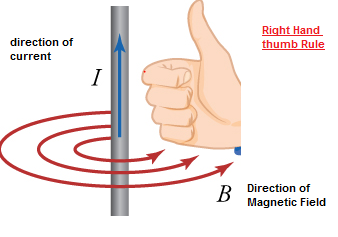# Problem solving tricks-Right hand rules (Magnetic fields due to currents)

Right hand rule for cross product:-
Consider that is the cross product of two vectors a and i.e.,
c=a x b
If direction of vector a is along the x axis and that of b along y axis then direction of vector c could be find using right hand rule for cross products. With the thumb, index, and middle fingers at right angles to each other the first (index) finger can represent a, the first vector in the product; the second (middle) finger, b, the second vector; and the thumb, c, the product. So direction of vector is perpendicular to the plane containing vectors a and b. This rule can be used in determining the directions of torque and angular momentum vectors and also to find the direction of the force on current carrying wire in an magnetic field.

Right hand thumb rule:-
This rule is used in situations when rotation is specified by a vector and we need to relate a curled element and a straight element , many such situations arises when you study magnetism. This can be done when you curl your fingers and straighten the thumb of your right hand. As an example you can consider an electric current passes through a straight wire. Here, the thumb points in the direction of the conventional current (from positive to negative), and the fingers point in the direction of the magnetic lines of flux.Related Articles

Comparison of Magnetic Force and electric forces Between two Moving Charges

Electric and Magnetic Fields

Electromagnetism

Electro Magnetism and Relatively

What are magnetic properties of matter